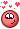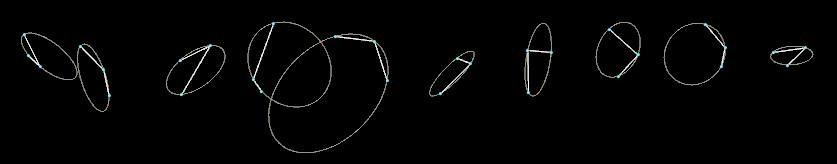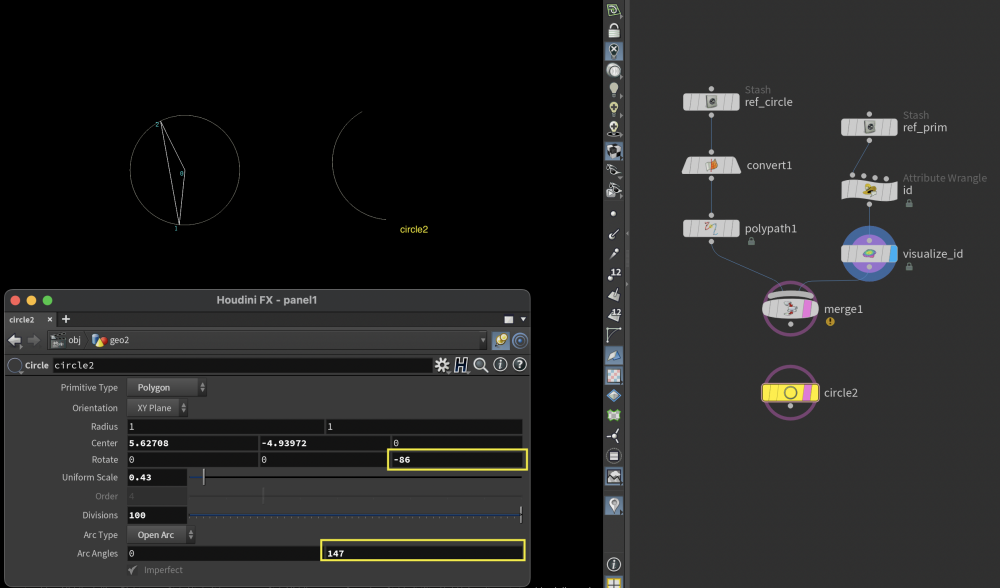## Recommended Posts

Please, do you have a vex code for creating Arc from 3 points?
From time to time it would be useful to have it.

AndrewVK shared this C++ source here:
https://www.sidefx.com/forum/topic/19104/?page=1#post-89849

I could rewrite it as an exercise and then I will share it ... but if anybody has it done, it would be a great time saver.

##### Share on other sites

HeavenMaybe it helps!

Edited by Librarian
•1

##### Share on other sites

Tesan, thank you very much for your hip file! Please, do you anybody have also a solution for a circular arc?

Here is a screenshot from the file, for a future reference:

And the complete wrangle is:

```// Convert the umap range from 0-1 to 0-1-0
float curveToHalf(
float value;
) {

return clamp(value, 0, 0.5) * clamp(fit(value, 0, 1, 1, 0) , 0, 0.5) * 4;
}
// Complement function
float complement(
float value;
) {

return 1 + (value * -1);
}
// Main interpolation function
float interpolate_curve(
float value;
string sign;
) {

float pi_half = 1.57079632679;

value = cos(value * pi_half);

if ( sign == "-" )
return 1 + (value * -1);
else
return value;

}
// Get user parameters from the UI
string  sign            = ch("sign");
int             complement      = int(ch("complement"));
int             curve_half      = int(ch("curve_half"));

// Create our classic umap attribute ranging over the points from 0-1
float   umap = float(@ptnum) / (npoints(@OpInput1) - 1);

// Convert the umap range
if ( curve_half == 1)
umap = curveToHalf(umap);

// Do the complement for the umap
if ( complement == 1)
umap = complement(umap);

// Interpolate curve based on the parameters
@P.y = interpolate_curve(umap, sign);```

##### Share on other sites

I have 100 % somewhere more files ..just need to find..and those  vex codes don't know Owner•1

##### Share on other sites

Hi,

I've found an older file. Here is a modification of it.

three_point_arc.hipnc

•1
•1

##### Share on other sites

Hi Jiri,

to get started I just wrote a script that turns a bunch of lines with three points into circles:It's basically calculating the intersection point and radius of rectangular vectors starting from the lines' midpoints. To be run in a primitive wrangle:

```// 3D INTERSECTION
// https://stackoverflow.com/questions/10551555/need-an-algorithm-for-3d-vectors-intersection
function vector intersection(vector r1, r2, e1, e2){
float u = dot(e1, e2);
float t1 = dot(r2 - r1, e1);
float t2 = dot(r2 - r1, e2);
float d1 = (t1 - u * t2) / (1 - u * u);
float d2 = (t2 - u * t1) / (u * u - 1);
vector p1 = r1 + e1 * d1;
vector p2 = r2 + e2 * d2;
vector pos_center = (p1 + p2) * 0.5;
return pos_center;
}

// INPUT POSITIONS
int pt_0 = primpoint(0, i@primnum, 0);
int pt_1 = primpoint(0, i@primnum, 1);
int pt_2 = primpoint(0, i@primnum, 2);

vector pos_A = point(0, 'P', pt_0);
vector pos_B = point(0, 'P', pt_1);
vector pos_C = point(0, 'P', pt_2);

vector mid_AB = (pos_A + pos_B) * 0.5;
vector mid_BC = (pos_B + pos_C) * 0.5;

// DIRECTIONS
vector dir_BA = normalize(pos_B - pos_A);
vector dir_BC = normalize(pos_B - pos_C);
vector dir_rect = normalize(cross(dir_BA, dir_BC));
vector dir_BA_rect = normalize(cross(dir_BA, dir_rect));
vector dir_BC_rect = normalize(cross(dir_BC, dir_rect));

vector pos_center = intersection(mid_AB, mid_BC, dir_BA_rect, dir_BC_rect);
matrix3 xform_circle = dihedral({0,0,1}, dir_rect);

// REMOVE INPUT GEOMETRY
removeprim(0, i@primnum, 1);```

circles_from_points.hipnc

•2
•2

##### Share on other sites

Is there a way to reverse engineer this so a Circle primitive rotates on the Z axis to point toward prim pt 1 and then work out the arc over to prim pt 2?

prim pt 0 is always the center of the circle.

I've mocked it up roughly here but the numbers aren't correct.arc_from_pts.hip

##### Share on other sites

If you take the first point as center, you can take the second point to define a radius (for example). The third point should have the same distance from center as the second point, or the arc will not intersect with this point.

You can derive the angle and the rotation axis from both direction (P1 - P0) and (P2 - P0). Now you can just rotate the first point along the angle.

If you a have guide axis, you can check if the rotation axis is conform (if not -> axis need to be inverted and the angle should be subtracted from 2*PI (360 degrees))

arc_by_center_two_other_points.hipnc

•1

##### Share on other sites

Thanks @Aizatulin That's really helpful in understanding!

•1

## Create an account

Register a new account